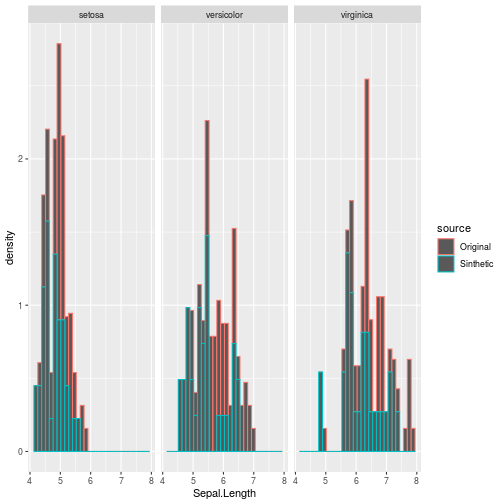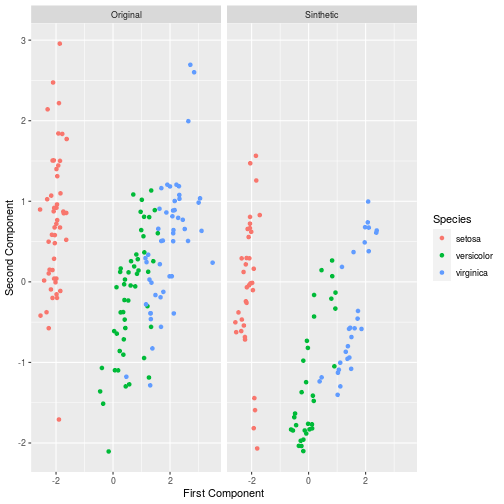## The AugmenterR Package

Machine learning techniques prove to be interesting and useful for many different problems, classification specially is a task where given a set of features a sample X must be labelled into a label Y

To do so a model must learn the conditional distribution P(Y|X) from the data, however when the number of available samples is small the learned models usually lack generalization capability

Given this here we present AugmenterR, the package is founded on a method based on conditional probability itself to generate novel samples, in the course of this document we present the two main functions an user will be interested in.

## Functions and Examples

Generate

This function should mostly be used in scenarios where the interest of the user is mostly for regression tasks, as its normal use is as a step for our main function for creating data for classification. It has the following parameters

• data Dataframe with the data we want to extend

• regression: Default value false returns a list containing a novel sample as well as some information that the method uses to revalidate a sample, If you desire to generate samples who are not conditioned to a class for regression use regression=TRUE

the following is an example of how to use it

``````require(AugmenterR)
NovelSample=Generate(iris,regression=TRUE)
print(NovelSample)
#>                     V1       V2 V3 V4   V5
#> X1.ncol.data. 4.373172 2.663216 NA NA <NA>
``````

Here we see NovelSample as a sample generated from the iris dataset which respects its distribution, we present these properties later using the function that conditions on a class

GenerateMultipleCandidates:

This function creates many novel samples by conditioning then on a class of interest, this can be useful for both working with imbalanced datasets as we can balance the classes generating novel samples as well as augmenting all classes on small data problems. We present how to run the function as well as present how the novel samples compare to the old ones

``````require(AugmenterR)
Setosa=GenerateMultipleCandidates(iris,'setosa',5,0.9,40)
Virginica=GenerateMultipleCandidates(iris,'virginica',5,0.9,40)
Versicolor=GenerateMultipleCandidates(iris,'versicolor',5,0.9,40)

#>                Sepal.Length Sepal.Width Petal.Length Petal.Width Species
#> X1.ncol.data.      4.974765    3.427988     1.332050         0.3  setosa
#> X1.ncol.data.1     5.020657    3.462028     1.525627         0.2  setosa
#> X1.ncol.data.2     4.289510    2.905976     1.299071         0.2  setosa
#> X1.ncol.data.3     4.606467    3.292332     1.228838         0.2  setosa
#> X1.ncol.data.4     5.109861    3.346060     1.340898         0.2  setosa
#> X1.ncol.data.5     4.427231    2.837835     1.336770         0.2  setosa
``````

To present how the synthetic samples and novel samples come from the same distribution we show some comparisons between then: First histograms

``````df=data.frame(iris,source='Original')
Setosa=data.frame(Setosa,source='Sinthetic')
Virginica=data.frame(Virginica,source='Sinthetic')
Versicolor=data.frame(Versicolor,source='Sinthetic')
df=rbind(df,Setosa,Virginica,Versicolor)
require(ggplot2)
ggplot2::ggplot(df) + aes(x=Sepal.Length,col=source) + facet_wrap(~Species) + geom_histogram(aes(y=..density..) )
#> `stat_bin()` using `bins = 30`. Pick better value with `binwidth`.
``````Using PCA

``````pc=prcomp(df[,1:4],center=TRUE,scale=TRUE)\$x

pc=data.frame(pc,df[,5:6])

ggplot2::ggplot(pc) + aes(x=pc[,1],y=pc[,2],col=Species) +facet_wrap(~source) + labs(x='First Component',y='Second Component') + geom_point()
``````